Vector Calculus MCQ Level - 2

# Vector Calculus MCQ Level - 2

Test Description

## 10 Questions MCQ Test Topic wise Tests for IIT JAM Physics | Vector Calculus MCQ Level - 2

Vector Calculus MCQ Level - 2 for IIT JAM 2023 is part of Topic wise Tests for IIT JAM Physics preparation. The Vector Calculus MCQ Level - 2 questions and answers have been prepared according to the IIT JAM exam syllabus.The Vector Calculus MCQ Level - 2 MCQs are made for IIT JAM 2023 Exam. Find important definitions, questions, notes, meanings, examples, exercises, MCQs and online tests for Vector Calculus MCQ Level - 2 below.
Solutions of Vector Calculus MCQ Level - 2 questions in English are available as part of our Topic wise Tests for IIT JAM Physics for IIT JAM & Vector Calculus MCQ Level - 2 solutions in Hindi for Topic wise Tests for IIT JAM Physics course. Download more important topics, notes, lectures and mock test series for IIT JAM Exam by signing up for free. Attempt Vector Calculus MCQ Level - 2 | 10 questions in 45 minutes | Mock test for IIT JAM preparation | Free important questions MCQ to study Topic wise Tests for IIT JAM Physics for IIT JAM Exam | Download free PDF with solutions
 1 Crore+ students have signed up on EduRev. Have you?
Vector Calculus MCQ Level - 2 - Question 1

### The line integral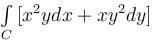taken along the closed path formed by y = x and x2 = y3 in the first quadrant will be valuated to :

Detailed Solution for Vector Calculus MCQ Level - 2 - Question 1

By Green's theorem, we have,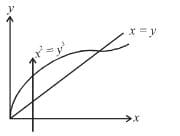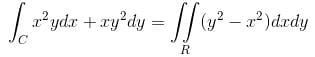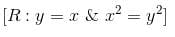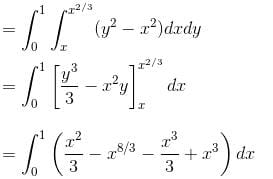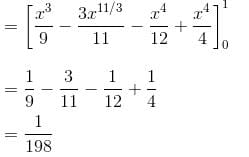Vector Calculus MCQ Level - 2 - Question 2

###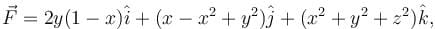where S : x2 + y2 + z2 = 1, z ≥ 0 and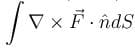will have the value :

Detailed Solution for Vector Calculus MCQ Level - 2 - Question 2

By Stoke’s Theorem,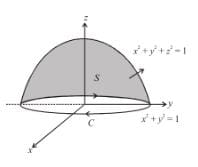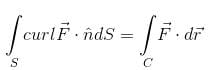where C : x2 + y2 = 1, z = 0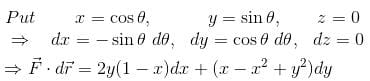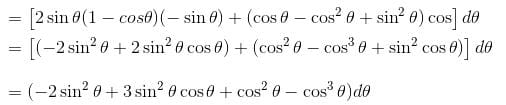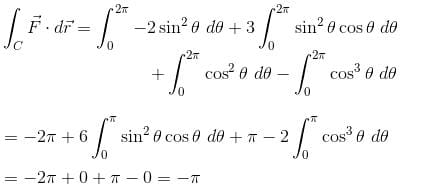Vector Calculus MCQ Level - 2 - Question 3

### The line integral of the vector field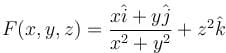along the helix defined by x = cos t, y = sin t,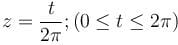is equal to :

Detailed Solution for Vector Calculus MCQ Level - 2 - Question 3

We have,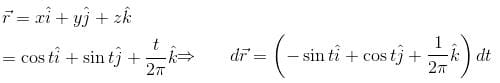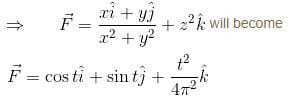Hence, the line integral of the given vector field will be,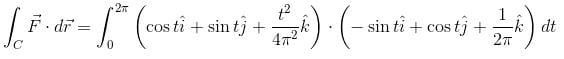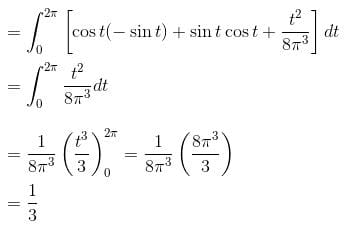Vector Calculus MCQ Level - 2 - Question 4

If  u = x + y + z, v = x2 + y2 + z2  and w = yz = zx + xy then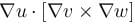is equal to :

Detailed Solution for Vector Calculus MCQ Level - 2 - Question 4

u = x + y + z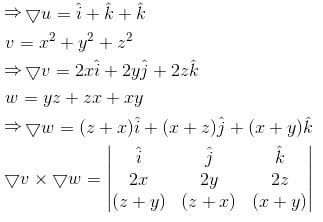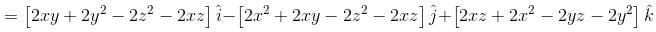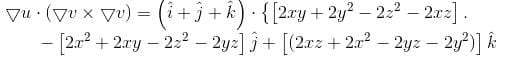= 0

Vector Calculus MCQ Level - 2 - Question 5

The potential function for the vector field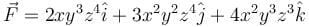will be :

Detailed Solution for Vector Calculus MCQ Level - 2 - Question 5

Compare the given vector field with the gradient of some function, say φ, i.e.,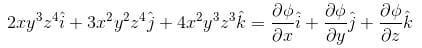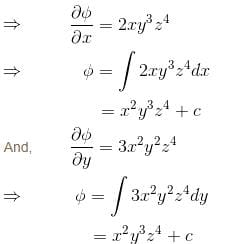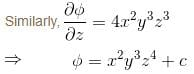⇒ The potential function for the given vector field would be x2y3z4.

Vector Calculus MCQ Level - 2 - Question 6

The value of the surface integral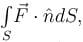where S is the closed surface of the solid bounded by the graphs of x = 4 and z = 9 – y2 and coordinate planes &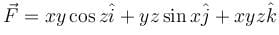will be given by :

Detailed Solution for Vector Calculus MCQ Level - 2 - Question 6

The given surface is z + y2 = 9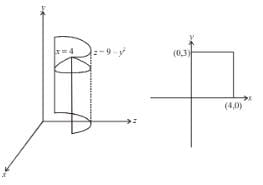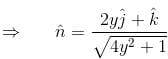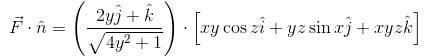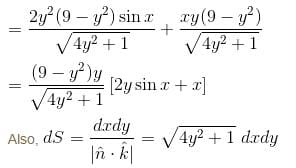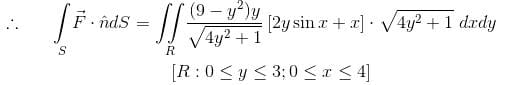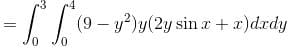The correct answer is: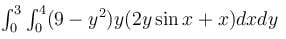Vector Calculus MCQ Level - 2 - Question 7

The value of the integral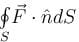where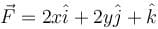and where S is the entire surface of the  paraboloid z = 1 - x2 - y2 with z = 0 together with the disk {(x, y) : x2 + y2 < 1}

Detailed Solution for Vector Calculus MCQ Level - 2 - Question 7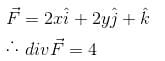By Gauss Divergence Theorem,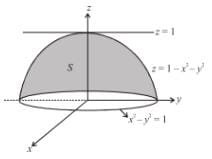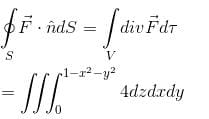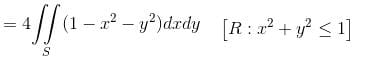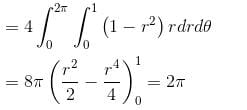Vector Calculus MCQ Level - 2 - Question 8

The value of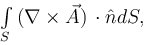where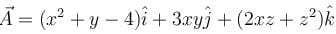and S is the surface of the paraboloid z = 4 – (x2 + y2) above the xy-plane will be :

Detailed Solution for Vector Calculus MCQ Level - 2 - Question 8

By Stokes theorem,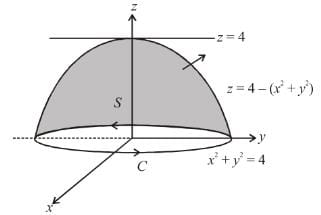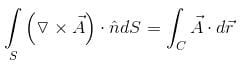Here, the boundary curve C of the surface will be given by x2 + y2 = 4, z = 0, or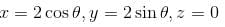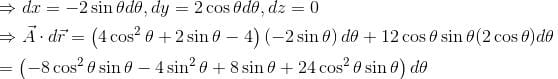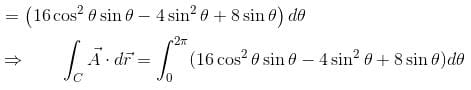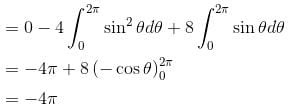Vector Calculus MCQ Level - 2 - Question 9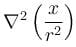is equal to :

Detailed Solution for Vector Calculus MCQ Level - 2 - Question 9

Consider,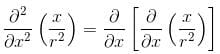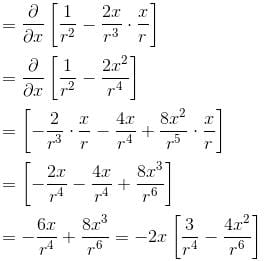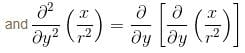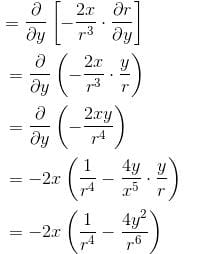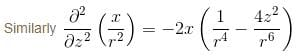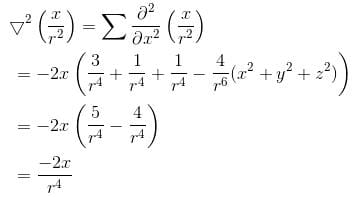The correct answer is: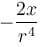Vector Calculus MCQ Level - 2 - Question 10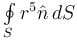is equivalent to :

Detailed Solution for Vector Calculus MCQ Level - 2 - Question 10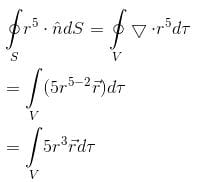The correct answer is: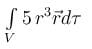## Topic wise Tests for IIT JAM Physics

217 tests
 Use Code STAYHOME200 and get INR 200 additional OFF Use Coupon Code
Information about Vector Calculus MCQ Level - 2 Page
In this test you can find the Exam questions for Vector Calculus MCQ Level - 2 solved & explained in the simplest way possible. Besides giving Questions and answers for Vector Calculus MCQ Level - 2, EduRev gives you an ample number of Online tests for practice

217 tests